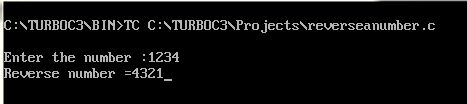UncleCoder.com

UncleCoder.com

Free programming examples and instructions

# C program to find Reverse of a Number

## C program for how to find Reverse of a Number

by Krishna

Posted on 27 Jun 2018 Category: C Views: 1218

C program to find Reverse of a Number

Here is a program to find the reverse of a given number. The below program is compiled successfully and the output for the same is given below.

``````#include <stdio.h>
#include <conio.h>
int main()
{
int n, reverse = 0, remainder;

printf("Enter the number: ");
scanf("%d", &n);

while(n != 0)
{
remainder = n%10;
reverse = reverse*10 + remainder;
n /= 10;
}

printf("Reverse Number = %d", reverse);
getch();
return 0;
}
``````

OUTPUT